GeeksforGeeks App
Open AppBrowser
Continue

# Split Pandas Dataframe by column value

Sometimes in order to analyze the Dataframe more accurately, we need to split it into 2 or more parts. The Pandas provide the feature to split Dataframe according to column index, row index, and column values, etc.

Let’ see how to Split Pandas Dataframe by column value in Python?

Now, let’s create a Dataframe:

villiers

## Python3

 `# importing pandas library``import` `pandas as pd` `# Initializing the nested list with Data-set``player_list ``=` `[[``'M.S.Dhoni'``, ``36``, ``75``, ``5428000``],``               ``[``'A.B.D Villiers'``, ``38``, ``74``, ``3428000``],``               ``[``'V.Kholi'``, ``31``, ``70``, ``8428000``],``               ``[``'S.Smith'``, ``34``, ``80``, ``4428000``],``               ``[``'C.Gayle'``, ``40``, ``100``, ``4528000``],``               ``[``'J.Root'``, ``33``, ``72``, ``7028000``],``               ``[``'K.Peterson'``, ``42``, ``85``, ``2528000``]]` `# creating a pandas dataframe``df ``=` `pd.DataFrame(player_list,``                  ``columns ``=` `[``'Name'``, ``'Age'``,``                             ``'Weight'``, ``'Salary'``])` `# show the dataframe``df`

Output: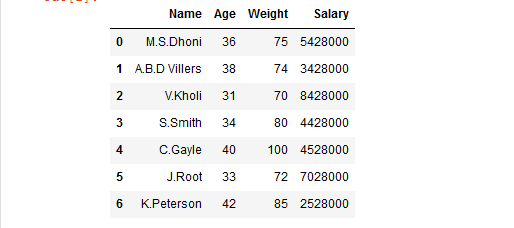Method 1: Using boolean masking approach.

This method is used to print only that part of dataframe in which we pass a boolean value True.

Example 1:

## Python3

 `# importing pandas library``import` `pandas as pd` `# Initializing the nested list with Data-set``player_list ``=` `[[``'M.S.Dhoni'``, ``36``, ``75``, ``5428000``],``               ``[``'A.B.D Villiers'``, ``38``, ``74``, ``3428000``],``               ``[``'V.Kholi'``, ``31``, ``70``, ``8428000``],``               ``[``'S.Smith'``, ``34``, ``80``, ``4428000``],``               ``[``'C.Gayle'``, ``40``, ``100``, ``4528000``],``               ``[``'J.Root'``, ``33``, ``72``, ``7028000``],``               ``[``'K.Peterson'``, ``42``, ``85``, ``2528000``]]` `# creating a pandas dataframe``df ``=` `pd.DataFrame(player_list,``                  ``columns ``=` `[``'Name'``, ``'Age'``,``                             ``'Weight'``, ``'Salary'``])` `# splitting the dataframe into 2 parts``# on basis of 'Age' column values``# using Relational operator``df1 ``=` `df[df[``'Age'``] >``=` `37``]` `# printing df1``df1`

Output: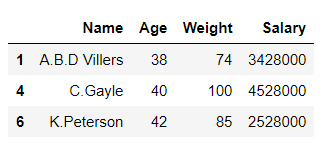## Python3

 `df2 ``=` `df[df[``'Age'``] < ``37``]` `# printing df2``df2`

Output: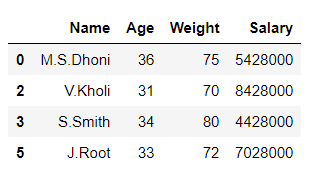In the above example, the data frame ‘df’ is split into 2 parts ‘df1’ and ‘df2’ on the basis of values of column ‘Age‘.

Example 2:

## Python3

 `# importing pandas library``import` `pandas as pd` `# Initializing the nested list with Data-set``player_list ``=` `[[``'M.S.Dhoni'``, ``36``, ``75``, ``5428000``],``               ``[``'A.B.D Villiers'``, ``38``, ``74``, ``3428000``],``               ``[``'V.Kholi'``, ``31``, ``70``, ``8428000``],``               ``[``'S.Smith'``, ``34``, ``80``, ``4428000``],``               ``[``'C.Gayle'``, ``40``, ``100``, ``4528000``],``               ``[``'J.Root'``, ``33``, ``72``, ``7028000``],``               ``[``'K.Peterson'``, ``42``, ``85``, ``2528000``]]` `# creating a pandas dataframe``df ``=` `pd.DataFrame(player_list,``                  ``columns ``=` `[``'Name'``, ``'Age'``,``                             ``'Weight'``, ``'Salary'``])` `# splitting the dataframe into 2 parts``# on basis of 'Weight' column values``mask ``=` `df[``'Weight'``] >``=` `80` `df1 ``=` `df[mask]` `# invert the boolean values``df2 ``=` `df[~mask]` `# printing df1``df1`

Output: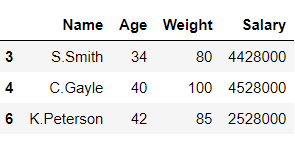## Python3

 `# printing df2``df2`

Output: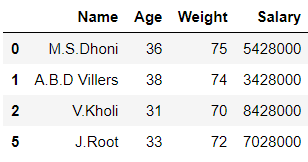In the above example, the data frame ‘df’ is split into 2 parts ‘df1’ and ‘df2’ on the basis of values of column ‘Weight‘.

Method 2: Using Dataframe.groupby().

This method is used to split the data into groups based on some criteria.

Example:

## Python3

 `# importing pandas library``import` `pandas as pd` `# Initializing the nested list with Data-set``player_list ``=` `[[``'M.S.Dhoni'``, ``36``, ``75``, ``5428000``],``               ``[``'A.B.D Villiers'``, ``38``, ``74``, ``3428000``],``               ``[``'V.Kholi'``, ``31``, ``70``, ``8428000``],``               ``[``'S.Smith'``, ``34``, ``80``, ``4428000``],``               ``[``'C.Gayle'``, ``40``, ``100``, ``4528000``],``               ``[``'J.Root'``, ``33``, ``72``, ``7028000``],``               ``[``'K.Peterson'``, ``42``, ``85``, ``2528000``]]` `# creating a pandas dataframe``df ``=` `pd.DataFrame(player_list,``                  ``columns ``=` `[``'Name'``, ``'Age'``,``                             ``'Weight'``, ``'Salary'``])` `# splitting the dataframe into 2 parts``# on basis of 'Salary' column values``# using dataframe.groupby() function``df1, df2 ``=` `[x ``for` `_, x ``in` `df.groupby(df[``'Salary'``] < ``4528000``)]` `# printing df1``df1`

Output: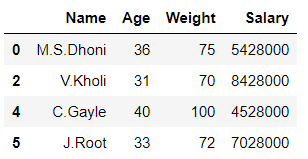## Python3

 `# printing df2``df2`

Output: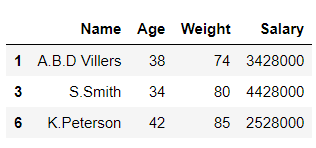In the above example, the data frame ‘df’ is split into 2 parts ‘df1’ and ‘df2’ on the basis of values of column ‘Salary‘.

My Personal Notes arrow_drop_up